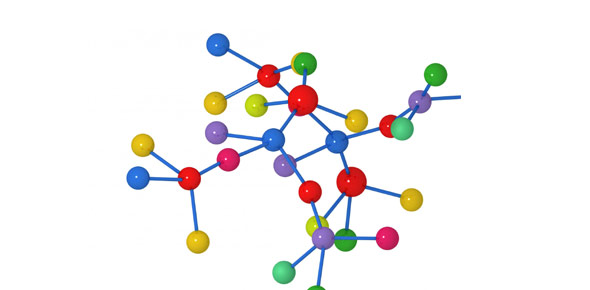# Chemhw4.2

8 QuestionsSettingsThese questions deal with percent composition and mole calculations. REMEMBER: period # followed by first and last name. you must score at least a 70!

Related Topics
• 1.
What is the percent composition by mass of nitrogen in NH4NO3 (gram-formula mass = 80.0 grams/mole)?
• A.

17.5%

• B.

35.0%

• C.

52.5%

• D.

60.0%

• 2.
In which compound is the percent composition by mass of chlorine equal to 42%?
• A.

HClO (gram-formula mass = 52 g/mol)

• B.

HClO2 (gram-formula mass = 68 g/mol)

• C.

HClO3 (gram-formula mass = 84 g/mol)

• D.

HClO4 (gram-formula mass = 100. g/mol)

• 3.
Determine the percent composition by mass of oxygen in the compound C6H12O6.    
• 4.
Some dry chemicals can be used to put out forest fires. One of these chemicals is NaHCO3. When NaHCO3(s) is heated, one of the products is CO2(g), as shown in the balanced equation below. 2NaHCO3 + heat  -------->  Na2CO3 + H2O + CO2 Calculate the percent composition by mass of carbon in the product Na2CO3.    
• 5.
What is the percent composition by mass of hydrogen in NH4HCO3 (gram-formula mass = 79 grams/mole)?
• A.

5.1%

• B.

6.3%

• C.

10.%

• D.

50.%

• 6.
A fluorescent light tube contains a noble gas and a drop of mercury. When the fluorescent light operates, the Hg is a vapor and there are free-flowing Hg ions and electrons in the tube. The electrons collide with Hg atoms that then emit ultraviolet (UV) radiation. The inside of the tube is coated with a mixture of several compounds that absorbs UV radiation. Ions in the coating emit a blend of red, green, and blue light that together appears as white light. The compound that produces red light is Y2O3. The compound that produces green light is CeMgAl11O19. The compound that produces blue light is BaMgAl10O17. Calculate the percent composition by mass of aluminum in the compound that produces green light.
• 7.
The percent composition by mass of nitrogen in NH4OH (gram-formula mass = 35 grams/mole) is equal to
• A.

4/35 × 100

• B.

14/35 × 100

• C.

35/14 × 100

• D.

35/4 × 100

• 8.
The sum of the atomic masses of the atoms in one molecule of C3H6Br2 is called the
• A.

Formula mass

• B.

Isotopic mass

• C.

Percent abundance

• D.

Percent composition